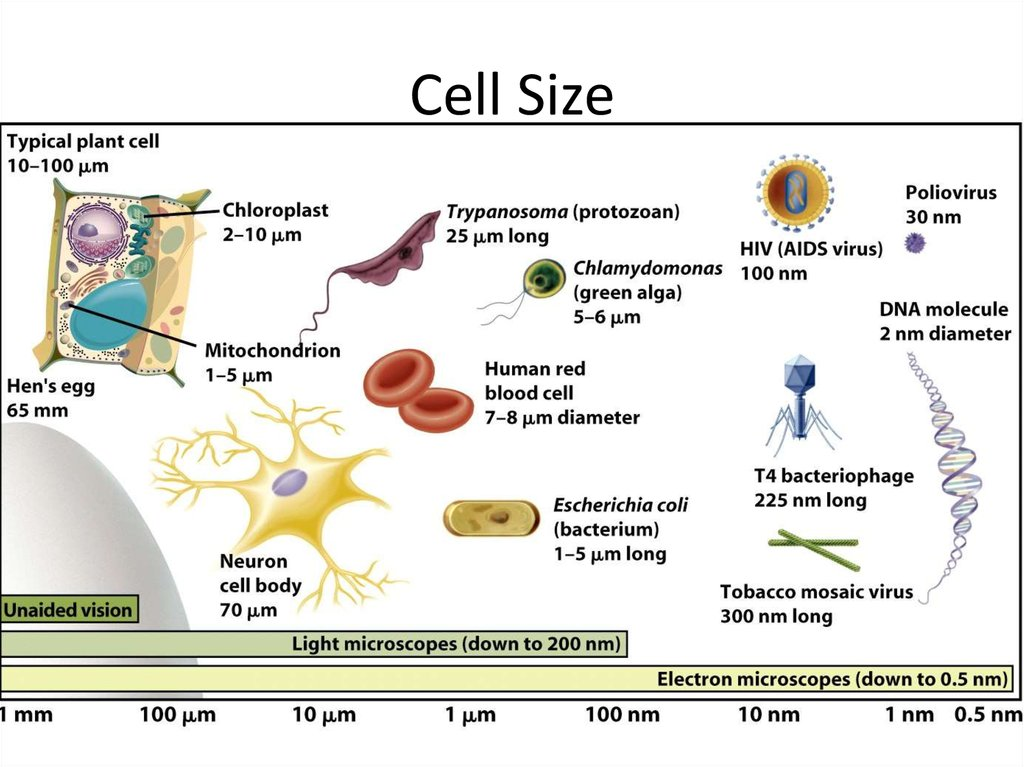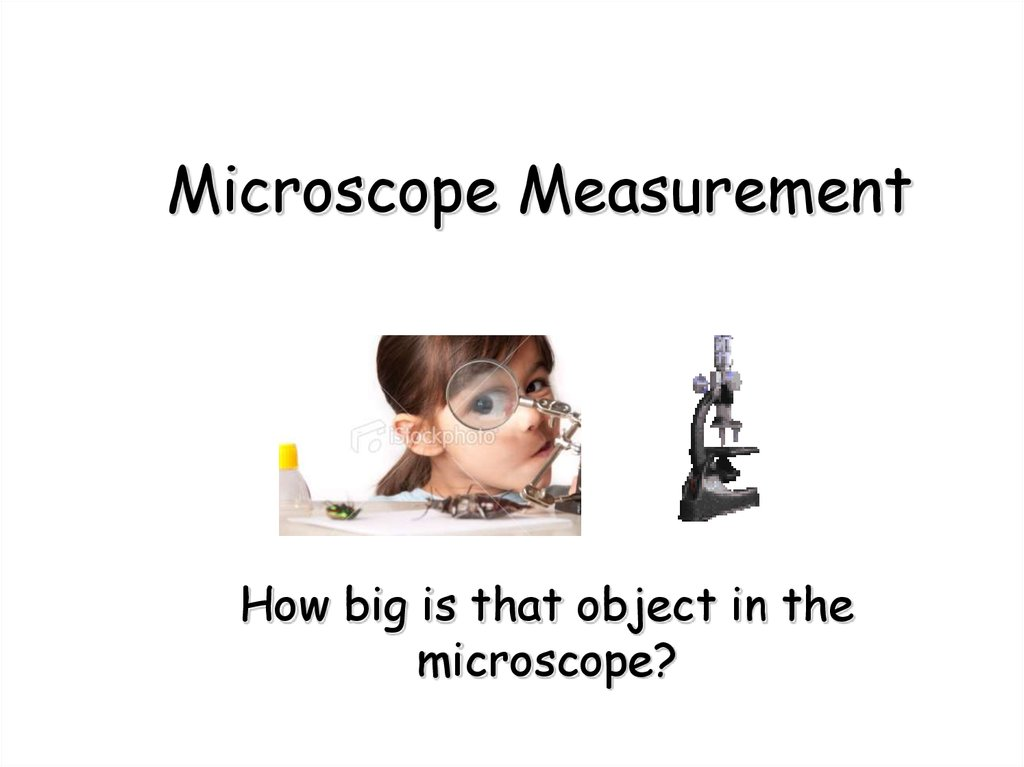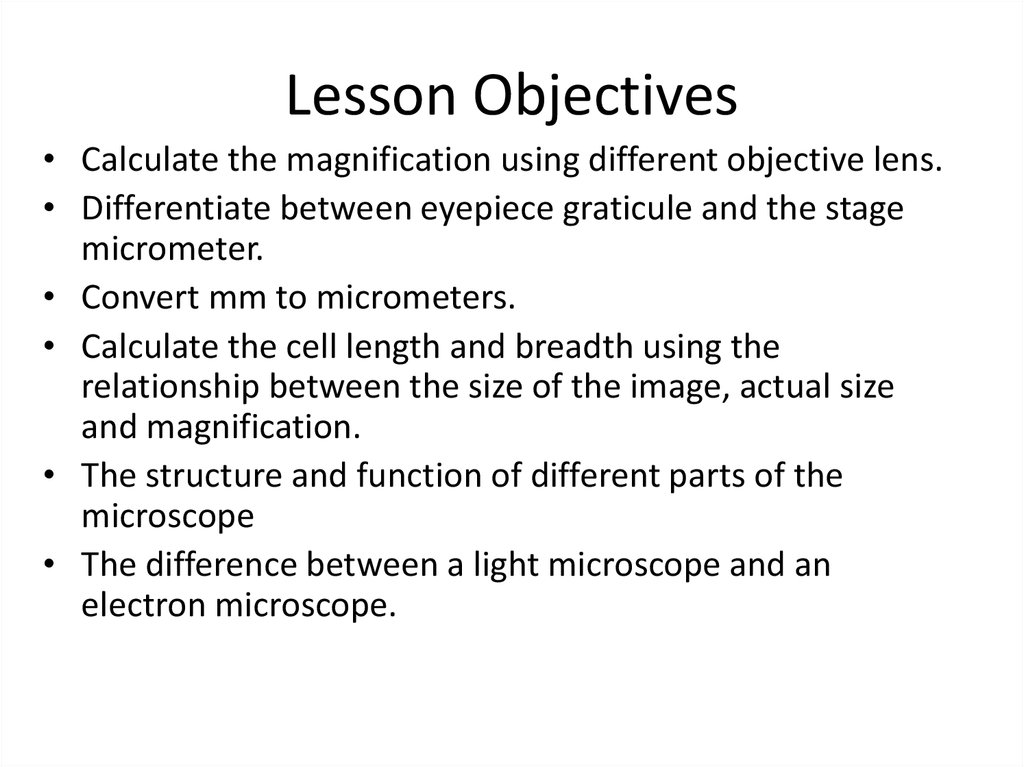# Microscope Measurement

## 2. Microscope Measurement

How big is that object in the
microscope?

## 3. Lesson Objectives

• Calculate the magnification using different objective lens.
• Differentiate between eyepiece graticule and the stage
micrometer.
• Convert mm to micrometers.
• Calculate the cell length and breadth using the
relationship between the size of the image, actual size
and magnification.
• The structure and function of different parts of the
microscope
• The difference between a light microscope and an
electron microscope.

## 6. Light Microscope

A light microscope (also, optical
microscope) is an optical
instrument used to make
objects larger in order to view
their details. It uses light to
illuminate the objects under
view

## 7. Electron Microscope

An electron microscope is an
optical instrument that uses a
beam of electrons to make
objects larger for a detailed
view

## 9. Light microscope vs Electron microscope

• What is the difference between a light
microscope and an electron microscope? A
number of differences such as the source of
light they use, their magnification level, cost,
resolving power, among other factors sets
these two types of microscopes apart from
each other.
• VIDEO

## 10.

What is happening to the image as
you increase the power of the
objective lens?

## 11. Calculating total magnification

If two lenses are always magnifying the
specimen, how do you figure out the
total magnification being used ?
Total Magnification = ocular x objective
= 10 x 4 (low power)
= 40 (low power)

## 12. How do we find the overall magnification of a light microscope?

Eyepiece
Objective lens
Eyepiece
Objective
Magnification Magnification
Overall
Magnification
X10
X4
40
X10
X10
100
X10
X40
400
X10
X100
1000

mm
1000
1000
Micrometre
1000
1000
nm

Nanometre
Micrometre
Millimetre
5
1
0.005
0.000005
0.001
0.000001
1000
1
0.001
1 000 000
1000
1
3000
3
0.003
0.007
0.000007
500
0.5
7
500 000

## 16.

The diagram below is a drawing of an organelle from a ciliated cell as seen
with an electron microscope.
A
B
Calculate the actual length of the organelle as shown ×by
the line AB in the
20 000
mm
I
102mm
A=
5.1
M =
20000
=
102000mm
20000

## 17. Calculating actual size:

Size of the magnified image > actual size

## 19. Field of View

When you look into a microscope, the “field of view” is the visible
circular area.
What happens to your field of view when you increase the power
of the objective lens?
By knowing the size of the field of view (diameter), you can
measure the size of objects in the microscope.
The size of objects in the field of view is different at each
magnification you have to calculate the diameters of the fields of
view at each magnification.
This process is called “calibrating your microscope”

## 20. Estimating Specimen Size

The area of the slide that you see when you
look through a microscope is called the "Field
of View".
If you know how wide your field of view is, you
can estimate the size of things you see in the
field of view.

## 22. Eye piece graticule or reticule

• It is a glass or plactic disc
with 8 divisions etched on
to its surface and fitted into
one eyepiece.
• The size of the eyepiece
reticule is constant despite
the change in magnification
of the object.
• The value of each division
varies with the change in
magnification.

## 23. Stage Micrometer

• simply a microscope slide
with a finely divided scale
marked on the surface.
• 1 division= 0.01 mm
• 10 divisions= 0.1 mm
• 100 divisions = 1 mm
• 1 mm = 1000 micrometers.

## 24. Instructions

Take sample of onion cell (peel of the onion)
Cover the subject glass with cover slip
Fix it with the stage clips.
Focus the specimen on low power objective lens.
Now change to medium power objective lens and
observe.
• Change to high power objective lens and observe.

## 25. IMPORTANT FORMULA!

Object Size = field of view (in mm) • 1000
number of “fits”
Object Size =
?
µm
** Remember that the field of view changes with
each objective!.